• 网上看到的一段很好的解释标识符控制的分水岭分割算法的实现，步骤注释都非常全面，就把精华部分保存了下来，侵删%% %步骤一：读取彩色图像并转化为灰度图 rgb = imread('coinseg.jpg'); I = rgb2gray(rgb); ...
网上看到的一段很好的解释标识符控制的分水岭分割算法的实现，步骤注释都非常全面，就把精华部分保存了下来，侵删%%

%步骤一：读取彩色图像并转化为灰度图
I = rgb2gray(rgb);

%如果原图中，目标物体是较暗的，即与假设相反，这里就要取反了
Fan=ones(size(I,1),size(I,2))*255;
Fan=uint8(Fan);
Fan=Fan-I;
I=Fan;

imshow(I), title('原图I')
%%
%******************步骤二：基于重建的开闭操作************************************

se = strel('disk',20);%disk指定构建一个圆形的结构体，第二个参数指定结构体的半径

%接下来，进行基于重建的开操作。使用imerode和imreconstruct函数实现
Ie = imerode(I, se);%先腐蚀‘erosion’
Iobr = imreconstruct(Ie, I);%再重建

Iobrd = imdilate(Iobr, se);%在基于重建的开操作的结果基础上，进行腐蚀
Iobrcbr = imreconstruct(imcomplement(Iobrd), imcomplement(Iobr));%重建，标记图像为腐蚀后图像取补，模板为腐蚀前原图取补。
Iobrcbr = imcomplement(Iobrcbr);%重建结果再取补，得到实际基于重建的闭操作的结果。
figure
imshow(Iobrcbr), title('基于重建的开+闭操作 (Iobrcbr)')

%%
%******************步骤三：标记前景物体************************************
%计算Iobrcbr的区域极大值来得到好的前景标记。
%得到的前景标记图fgm是二值图，白色对应前景区域
fgm = imregionalmax(Iobrcbr);

%为了方便解释结果，将前景标记图叠加到原始图像上
I2 = I;
I2(fgm) = 255;%将fgm中的前景区域（像素值为1）标记到原图上（置白色）

%注意到一些大部分重合或被阴影遮挡的物体没有被标记出来。这意味着这些物体最终可能不会被正确的分割出来。
%并且，有些物体中前景标记正确的到达了物体的边缘。这意味着你应该清除掉标记斑块的边缘，向内收缩一点。
%你可以通过先闭操作，再腐蚀做到这点。
se2 = strel(ones(5,5));
fgm2 = imclose(fgm, se2);
fgm3 = imerode(fgm2, se2);

%这个操作会导致遗留下一些离群的孤立点，这些是需要被移除的。
%你可以通过bwareaopen做到这点，函数将移除那些包含像素点个数少于指定值的区域。
fgm4 = bwareaopen(fgm3, 20);
I3 = I;
I3(fgm4) = 255;

%%
%*********************第四步：计算背景标记**********************************
%本例中设计出的标记背景算法的前提假设是：图像中相对亮的是物体，相对暗的区域是背景。
%如果不满足这条假设，标记结果可能不甚理想

%现在你需要标记背景。在去除噪点后的图像Iobrcbr中，暗像素属于背景，所以你可以先进行一下阈值操作。
%bw = imbinarize(Iobrcbr);
bw=im2bw(Iobrcbr,graythresh(Iobrcbr));%我使用上一行代码报错了，故换了一种二值化方法

%背景像素现在是黑的了，但是理想情况下我们不希望背景标记太接近我们想要分割的物体的边界。

D = bwdist(bw);
%D = bwdist(BW)计算二值图像BW的欧几里得矩阵。对BW的每一个像素，距离变换指定像素和最近的BW非零像素的距离。
%bwdist默认使用欧几里得距离公式。BW可以由任意维数，D与BW有同样的大小。

%由于bw中目标物体是白色的1.所以D中对应的目标物体处均是0，随着进入背景越深，对应像素值越大。
%这时正好符合我们使用分水岭算法的假设（想分出的目标物体数值较低）
%于是得到的背景标记是 物体与背景间的一个圈，能够包住目标物体
DL = watershed(D);
bgm = DL == 0;%分水岭变换结果L中，同一区域用同一数字表示，区域间分界线同一由0标识

%%
%******************第五步：计算分割函数（修改后）的分水岭变换*****************
% 函数imimposemin可以被用来修改一副图片，使得其只在指定的位置处取得局部最小值
% 这里你可以使用imimposemin来修改梯度幅值图像，使得局部最小值只出现在前景标记和背景标记处。
% 从结果来看imimposemin会将指定区域置为-Inf，从而成为极小值
% 且图像变得相当“平整”，一块块的相同数值的区域

%使用Sobel边缘检测算子，imfilter函数，和一些简单的四则运算来计算梯度幅值。
%梯度值总是在物体的边缘处高，而总是在物体内部低。
hy = fspecial('sobel');%获取sobel算子模板，计算纵向梯度
hx = hy';%横向模板
Iy = imfilter(double(I), hy, 'replicate');%计算纵向梯度
Ix = imfilter(double(I), hx, 'replicate');

%Finally we are ready to compute the watershed-based segmentation.

%%
%******************第六步：可视化结果************************************
I4 = I;
I4(imdilate(L == 0, ones(3, 3)) | bgm | fgm4) = 255;
figure
imshow(I4)
title('在原图上绘制前景、背景标记，以及分割边界')

Lrgb = label2rgb(L, 'jet', 'w', 'shuffle');
figure
imshow(Lrgb)
title('Colored watershed label matrix (Lrgb)')

figure
imshow(I)
hold on
himage = imshow(Lrgb);
title('Lrgb superimposed transparently on original image')  
展开全文图像处理
• matlab实现图像分水岭分割算法并且有改进的算法实现，两者相互比较效果，并且文档最后直接复制了全部的实现代码
• matlab分水岭分割算法实例，内涵有实测数据，亲测可用！
• 该程序使用matlab完成的分水岭算法，很具有代表性，程序很好，很实用
• 应用标记符控制分水岭分割 有效解决了分水岭算法在图像分割中的过分割问题
• 数字图像处理 图像分割分水岭算法 源代码 matlab
• 1. 分水岭分割方法它是依赖于形态学的，图像的灰度等级不一样，如果图像的灰度等级一样的情况下怎么人为的把它造成不一样？可以通过距离变换实现，这样它们的灰度值就有了阶梯状的变换。风水岭算法常见的有三种方法...
1. 分水岭分割方法它是依赖于形态学的，图像的灰度等级不一样，如果图像的灰度等级一样的情况下怎么人为的把它造成不一样？可以通过距离变换实现，这样它们的灰度值就有了阶梯状的变换。风水岭算法常见的有三种方法：(1)基于浸泡理论的分水岭分割方法；(2)基于连通图方法；(3)基于距离变换的方法。OpenCV 中是基于距离变换的分割方法，就相当于我们的小山头(认为造成的)。基本的步骤：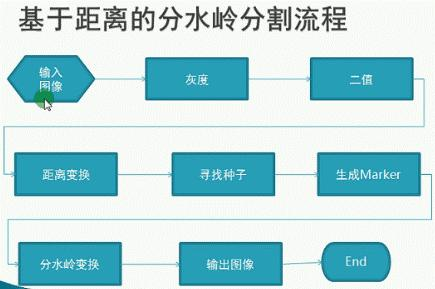例子1 粘连对象分离和计数。例子代码：#include#includeusing namespace std;using namespace cv;void test(){    Mat srcImg;    srcImg = imread("pill_002.png");    if (srcImg.empty())    {        cout <>contours;    //找到 marker 的轮廓    findContours(distMaskImg, contours, RETR_EXTERNAL,                 CHAIN_APPROX_SIMPLE, Point(0, 0));    //create marker 填充 marker    Mat  markersImg = Mat::zeros(srcImg.size(), CV_32SC1);    for (int i = 0; i (i),                     Scalar::all(static_cast(i)+1), -1);     }    circle(markersImg, Point(5, 5), 3, Scalar(255), -1);    //形态学操作 - 彩色图像，目的是去掉干扰，让结果更好。    Mat kernel = getStructuringElement(MORPH_RECT, Size(3, 3), Point(-1, -1));    morphologyEx(srcImg, srcImg, MORPH_ERODE, kernel);    //完成分水岭变换    watershed(srcImg, markersImg);    Mat mark = Mat::zeros(markersImg.size(), CV_8UC1);    markersImg.convertTo(mark, CV_8UC1);    bitwise_not(mark, mark, Mat());    namedWindow("watershed", CV_WINDOW_AUTOSIZE);    imshow("watershed", mark);    //下面的步骤可以不做，最好做出来让结果显示更美观。    //生成随机颜色    vectorcolors;    for (int i = 0; i (i, j);            if (index > 0 && index <= contours.size())            {                dstImg.at(i, j) = colors[index - 1];            }            else            {                dstImg.at(i, j) = Vec3b(0, 0, 0);            }        }    }    cout <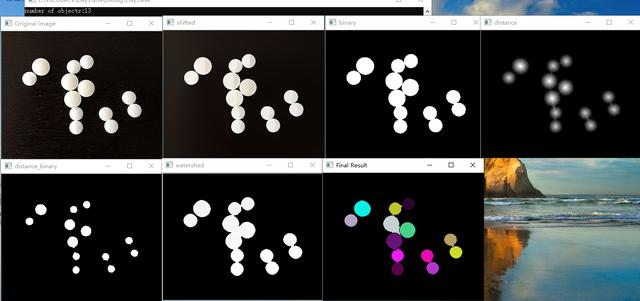总结：有时候会导致碎片化，过度分割，因为二值化中如果有很多小的黑点或碎片，在分割的时候导致很多 mask ，即小山头太多了，这个时候我们要考虑怎么去合并它，可以通过联通区域的直方图，或者像素值均值相似程度等。例子2：图像分割#include#includeusing namespace std;using namespace cv;//执行分水岭算法函数Mat watershedCluster(Mat &srcImg, int &numSegments);//结果显示函数void DisplaySegments(Mat &markersImg, int numSegments);void test(){    Mat srcImg;    srcImg = imread("toux.jpg");    if (srcImg.empty())    {        cout <>contours;    vectorhireachy;    findContours(distImg, contours, hireachy, RETR_CCOMP, CHAIN_APPROX_SIMPLE);    if (contours.empty())    {        return Mat();    }    Mat markersImg(distImg.size(), CV_32S);    markersImg = Scalar::all(0);    for (int i = 0; i colors;    for (int i = 0; i (i, j);            if (index > 0 && index <= numSegments)            {                dstImg.at(i, j) = colors[index - 1];            }            else            {                dstImg.at(i, j) = Vec3b(255, 255, 255);            }        }    }    cout <效果图：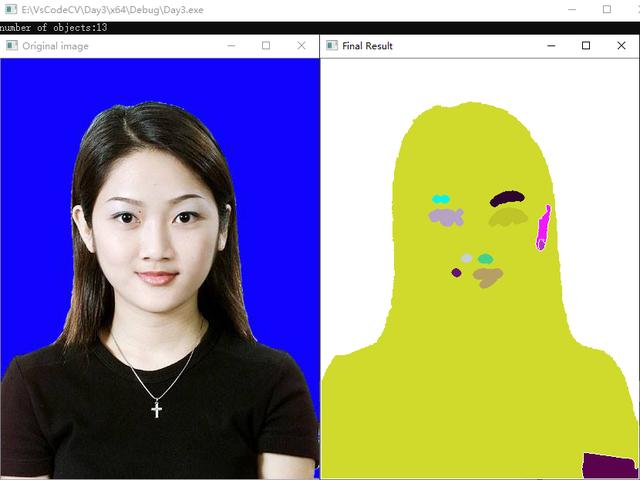2. GrabCut 算法分割图像GrabCut 算法的原理前面有介绍过，这里就不在介绍了，具体可以看下文章末尾往期推荐中阅读。下面例子实现图像中对象的抠图。基本步骤：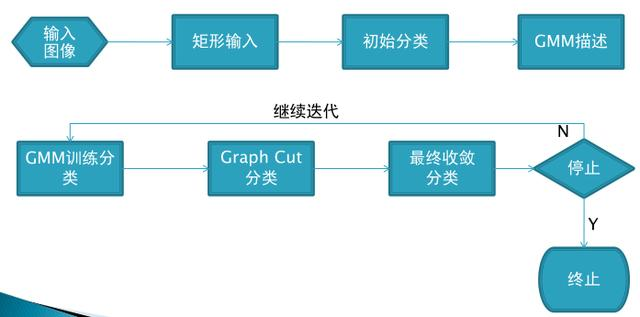例子代码：#include#includeusing namespace std;using namespace cv;int numRun = 0; //算法迭代次数bool init = false;Rect rect;Mat srcImg, MaskImg, bgModel, fgModel;//鼠标回调函数void onMouse(int event, int x, int y, int flags, void* param);void showImg();  //显示画的图片void setRoiMask();  //选择 ROI 的函数void runGrabCut();  //执行算法函数static void ShowHelpText();  //提示用户操作函数void test(){    srcImg = imread("toux.jpg");    if (srcImg.empty())    {        cout < 1 && rect.height > 1)        {            showImg();        }        break;    default:        break;    }}void showImg(){    Mat result, binMask;    binMask.create(MaskImg.size(), CV_8UC1);    binMask = MaskImg & 1;    if (init)    {        srcImg.copyTo(result,binMask);    }    else    {        srcImg.copyTo(result);    }    rectangle(result, rect, Scalar(0, 0, 255), 2, 8);    namedWindow("Original image", CV_WINDOW_AUTOSIZE);    imshow("Original image", result);}void setRoiMask(){    //GC_BGD = 0   明确属于背景的像素    //GC_FGD = 1   明确属于前景的像素    //GC_PR_BGD = 2  可能属于背景的像素    //GC_PR_FGD = 3  可能属于前景的像素    MaskImg.setTo(GC_BGD);      //为了避免选择越界    rect.x = max(0, rect.x);    rect.y = max(0, rect.y);    rect.width = min(rect.width, srcImg.cols - rect.x);    rect.height = min(rect.height, srcImg.rows - rect.y);    //把我们选取的那一块设为前景    MaskImg(rect).setTo(Scalar(GC_PR_FGD));}void runGrabCut(){    if (rect.width 效果图：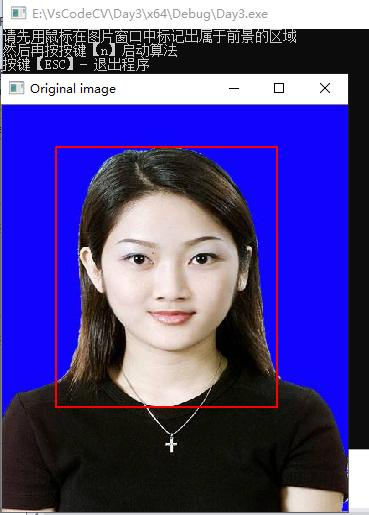展开全文• 基于MATLAB分水岭分割算法源代码 可以对图像进行分割处理
• 分水岭算法是现在医学图像分割的经典算法，具有非常高的参考价值
• 此处为用分水岭算法实现MATLAB的图像分割，在实际运用中有较强的实用价值
• 基于Matlab的标记分水岭分割算法　   (2011-05-21 13:57:31) 转载▼ 标签：  matlab   标记分水岭   分割   代码     lyqmath ...Separating touch


基于Matlab的标记分水岭分割算法(2011-05-21 13:57:31)转载▼

标签：

matlab

标记分水岭

分割

代码

lyqmath

http://blog.sina.com.cn/lyqmath

1 综述

Separating touching objects in an image is one of the more difficult image processing operations. The watershed transform is often applied to this problem. The watershed transform finds "catchment basins"(集水盆) and "watershed ridge lines"(山脊线) in an image by
treating it as a surface where light pixels are high and dark pixels are low.

如果图像中的目标物体是连接在一起的，则分割起来会更困难，分水岭分割算法经常用于处理这类问题，通常会取得比较好的效果。分水岭分割算法把图像看成一幅“地形图”，其中亮度比较强的区域像素值较大，而比较暗的区域像素值较小，通过寻找“汇水盆地”和“分水岭界限”，对图像进行分割。

Segmentation using the watershed transform works better if you can identify, or "mark," foreground objects and background locations. Marker-controlled watershed segmentation follows this basic procedure:

直接应用分水岭分割算法的效果往往并不好，如果在图像中对前景对象和背景对象进行标注区别，再应用分水岭算法会取得较好的分割效果。基于标记控制的分水岭分割方法有以下基本步骤：

1. Compute a segmentation function. This is an image whose dark regions are the objects you are trying to segment.

1.计算分割函数。图像中较暗的区域是要分割的对象。

2. Compute foreground markers. These are connected blobs of pixels
within each of the objects.

2.计算前景标志。这些是每个对象内部连接的斑点像素。

3. Compute background markers. These are pixels that are not part
of any object.

3.计算背景标志。这些是不属于任何对象的像素。

4. Modify the segmentation function so that it only has minima at
the foreground and background marker locations.

4.修改分割函数，使其仅在前景和后景标记位置有极小值。

5. Compute the watershed transform of the modified segmentation
function.

5.对修改后的分割函数做分水岭变换计算。

Use by Matlab Image Processing Toolbox

使用MATLAB图像处理工具箱

注：期间用到了很多图像处理工具箱的函数，例如fspecial、imfilter、watershed、label2rgb、imopen、imclose、imreconstruct、imcomplement、imregionalmax、bwareaopen、graythresh和imimposemin函数等。

2 步骤

Step 1: Read in the Color Image and Convert it to Grayscale

第一步：读入彩色图像，将其转化成灰度图像

clc; clear all; close all;

if ndims(rgb) == 3

I = rgb2gray(rgb);

else

I = rgb;

end

figure('units', 'normalized', 'position', [0 0 1 1]);

subplot(1, 2, 1); imshow(rgb); title('原图');

subplot(1, 2, 2); imshow(I); title('灰度图');Step 2: Use the Gradient Magnitude as the Segmentation Function

第2步：将梯度幅值作为分割函数

Use the Sobel edge masks, imfilter, and some simple arithmetic to compute the gradient magnitude. The gradient is high at the borders of the objects and low (mostly) inside the objects.

使用Sobel边缘算子对图像进行水平和垂直方向的滤波，然后求取模值，sobel算子滤波后的图像在边界处会显示比较大的值，在没有边界处的值会很小。

hy = fspecial('sobel');

hx = hy';

Iy = imfilter(double(I), hy, 'replicate');

Ix = imfilter(double(I), hx, 'replicate');

figure('units', 'normalized', 'position', [0 0 1 1]);

subplot(1, 2, 1); imshow(I,[]), title('灰度图像')Can you segment the image by using the watershed transform directly on the gradient magnitude?

可否直接对梯度幅值图像使用分水岭算法？

Lrgb = label2rgb(L);

figure('units', 'normalized', 'position', [0 0 1 1]);

subplot(1, 2, 2); imshow(Lrgb); title('梯度幅值做分水岭变换')No. Without additional preprocessing such as the marker computations below, using the watershed transform directly often results in "oversegmentation."

直接使用梯度模值图像进行分水岭算法得到的结果往往会存在过度分割的现象。因此通常需要分别对前景对象和背景对象进行标记，以获得更好的分割效果。

Step 3: Mark the Foreground Objects

第3步：标记前景对象

A variety of procedures could be applied here to find the foreground markers, which must be connected blobs of pixels inside each of the foreground objects. In this example you'll use morphological techniques called "opening-by-reconstruction" and "closing-by-reconstruction"
to "clean" up the image. These operations will create flat maxima inside each object that can be located using imregionalmax.

有多种方法可以应用在这里来获得前景标记，这些标记必须是前景对象内部的连接斑点像素。这个例子中，将使用形态学技术“基于开的重建”和“基于闭的重建”来清理图像。这些操作将会在每个对象内部创建单位极大值，使得可以使用imregionalmax来定位。

开运算和闭运算：先腐蚀后膨胀称为开；先膨胀后腐蚀称为闭。开和闭这两种运算可以除去比结构元素小的特定图像细节，同时保证不产生全局几何失真。开运算可以把比结构元素小的突刺滤掉，切断细长搭接而起到分离作用；闭运算可以把比结构元素小的缺口或孔填充上，搭接短的间隔而起到连接作用。

Opening is an erosion followed by a dilation, while opening-by-reconstruction is an erosion followed by a morphological reconstruction. Let's compare the two. First, compute the opening using imopen.

开操作是腐蚀后膨胀，基于开的重建（基于重建的开操作）是腐蚀后进行形态学重建。下面比较这两种方式。首先，用imopen做开操作。

se = strel('disk', 20);

Io = imopen(I, se);

figure('units',
'normalized', 'position', [0 0 1 1]);

subplot(1, 2, 1); imshow(I, []); title('灰度图像');

subplot(1, 2, 2); imshow(Io), title('图像开操作')Next compute the opening-by-reconstruction using imerode and imreconstruct.

接下来，通过腐蚀后重建来做基于开的重建计算。

Ie = imerode(I, se);

Iobr = imreconstruct(Ie, I);

figure('units', 'normalized', 'position', [0 0 1 1]);

subplot(1, 2, 1); imshow(I, []); title('灰度图像');

subplot(1, 2, 2); imshow(Iobr, []), title('基于开的重建图像')Following the opening with a closing can remove the dark spots and stem marks. Compare a regular morphological closing with a closing-by-reconstruction. First try imclose:

开操作后，接着进行闭操作，可以移除较暗的斑点和枝干标记。对比常规的形态学闭操作和基于闭的重建操作。首先，使用imclose：

Ioc = imclose(Io, se);

Ic = imclose(I, se);

figure('units', 'normalized', 'position', [0 0 1 1]);

subplot(2, 2, 1); imshow(I, []); title('灰度图像');

subplot(2, 2, 2); imshow(Io, []); title('开操作图像');

subplot(2, 2, 3); imshow(Ic, []); title('闭操作图像');

subplot(2, 2, 4); imshow(Ioc, []), title('开闭操作');Now use imdilate followed by imreconstruct. Notice you must complement the image inputs and output of imreconstruct.
IM2 = imcomplement(IM) computes the complement(补集) of the image IM. IM can be a binary, intensity, or RGB image. IM2 has the same class and size as IM.

现在使用imdilate，然后使用imreconstruct。注意必须对输入图像求补，对imreconstruct输出图像求补。IM2 = imcomplement(IM)计算图像IM的补集。IM可以是二值图像，或者RGB图像。IM2与IM有着相同的数据类型和大小。

Iobrd = imdilate(Iobr, se);

Iobrcbr = imreconstruct(imcomplement(Iobrd), imcomplement(Iobr));

Iobrcbr = imcomplement(Iobrcbr);

figure('units', 'normalized', 'position', [0 0 1 1]);

subplot(2, 2, 1); imshow(I, []); title('灰度图像');

subplot(2, 2, 2); imshow(Ioc, []); title('开闭操作');

subplot(2, 2, 3); imshow(Iobr, []); title('基于开的重建图像');

subplot(2, 2, 4); imshow(Iobrcbr, []), title('基于闭的重建图像');As you can see by comparing Iobrcbr with Ioc, reconstruction-based opening and closing are more effective than standard opening and closing at removing small blemishes without affecting the overall shapes of the objects. Calculate the regional maxima of Iobrcbr
to obtain good foreground markers.

通过比较Iobrcbr和loc可以看到，在移除小污点同时不影响对象全局形状的应用下，基于重建的开闭操作要比标准的开闭重建更加有效。计算Iobrcbr的局部极大来得到更好的前景标记。

fgm = imregionalmax(Iobrcbr);

figure('units', 'normalized', 'position', [0 0 1 1]);

subplot(1, 3, 1); imshow(I, []); title('灰度图像');

subplot(1, 3, 2); imshow(Iobrcbr, []); title('基于重建的开闭操作');

subplot(1, 3, 3); imshow(fgm, []); title('局部极大图像');To help interpret the result, superimpose(叠加) the foreground marker image on the original image.

为了帮助理解这个结果，叠加前景标记到原图上。

It1 = rgb(:, :, 1);

It2 = rgb(:, :, 2);

It3 = rgb(:, :, 3);

It1(fgm) = 255; It2(fgm) = 0; It3(fgm) = 0;

I2 = cat(3, It1, It2, It3);

figure('units', 'normalized', 'position', [0 0 1 1]);

subplot(2, 2, 1); imshow(rgb, []); title('原图像');

subplot(2, 2, 2); imshow(Iobrcbr, []); title('基于重建的开闭操作');

subplot(2, 2, 3); imshow(fgm, []); title('局部极大图像');

subplot(2, 2, 4); imshow(I2); title('局部极大叠加到原图像');Notice that some of the mostly-occluded and shadowed objects are not marked, which means that these objects will not be segmented properly in the end result. Also, the foreground markers in some objects go right up to the objects' edge. That means you should
clean the edges of the marker blobs and then shrink them a bit. You can do this by a closing followed by an erosion.

注意到大多闭塞处和阴影对象没有被标记，这就意味着这些对象在结果中将不会得到合理的分割。而且，一些对象的前景标记会一直到对象的边缘。这就意味着应该清理标记斑点的边缘，然后收缩它们。可以通过闭操作和腐蚀操作来完成。

se2 = strel(ones(5,5));

fgm2 = imclose(fgm, se2);

fgm3 = imerode(fgm2, se2);

figure('units', 'normalized', 'position', [0 0 1 1]);

subplot(2, 2, 1); imshow(Iobrcbr, []); title('基于重建的开闭操作');

subplot(2, 2, 2); imshow(fgm, []); title('局部极大图像');

subplot(2, 2, 3); imshow(fgm2, []); title('闭操作');

subplot(2, 2, 4); imshow(fgm3, []); title('腐蚀操作');This procedure tends to leave some stray isolated pixels that must be removed. You can do this using bwareaopen,
which removes all blobs that have fewer than a certain number of pixels. BW2 = bwareaopen(BW,P) removes from a binary image all connected components (objects) that have fewer than P pixels, producing another binary image, BW2.

这个过程将会留下一些偏离的孤立像素，应该移除它们。可以使用bwareaopen，用来移除少于特定像素个数的斑点。BW2 = bwareaopen(BW,P)从二值图像中移除所以少于P像素值的连通块，得到另外的二值图像BW2。

fgm4 = bwareaopen(fgm3, 20);

It1 = rgb(:, :, 1);

It2 = rgb(:, :, 2);

It3 = rgb(:, :, 3);

It1(fgm4) = 255; It2(fgm4) = 0; It3(fgm4) = 0;

I3 = cat(3, It1, It2, It3);

figure('units', 'normalized', 'position', [0 0 1 1]);

subplot(2, 2, 1); imshow(I2, []); title('局部极大叠加到原图像');

subplot(2, 2, 2); imshow(fgm3, []); title('闭腐蚀操作');

subplot(2, 2, 3); imshow(fgm4, []); title('去除小斑点操作');

subplot(2, 2, 4); imshow(I3, []); title('修改局部极大叠加到原图像');Step 4: Compute Background Markers

Now you need to mark the background. In the cleaned-up image, Iobrcbr, the dark pixels belong to the background, so you could start with a thresholding operation.

第4步：计算背景标记

现在，需要标记背景。在清理后的图像Iobrcbr中，暗像素属于背景，所以可以从阈值操作开始。

bw = im2bw(Iobrcbr, graythresh(Iobrcbr));

figure('units', 'normalized', 'position', [0 0 1 1]);

subplot(1, 2, 1); imshow(Iobrcbr, []); title('基于重建的开闭操作');

subplot(1, 2, 2); imshow(bw, []); title('阈值分割');The background pixels are in black, but ideally we don't want the background markers to be too close to the edges of the objects we are trying to segment. We'll "thin" the background by computing the "skeleton by influence zones", or SKIZ, of the foreground
of bw. This can be done by computing the watershed transform of the distance transform of bw, and then looking for the watershed ridge lines (DL == 0) of the result. D = bwdist(BW) computes the Euclidean distance transform of the binary image BW. For each
pixel in BW, the distance transform assigns a number that is the distance between that pixel and the nearest nonzero pixel of BW. bwdist uses
the Euclidean distance metric by default. BW can have any dimension. D is the same size as BW.

背景像素在黑色区域，但是理想情形下，不必要求背景标记太接近于要分割的对象边缘。通过计算“骨架影响范围”来“细化”背景，或者SKIZ，bw的前景。这个可以通过计算bw的距离变换的分水岭变换来实现，然后寻找结果的分水岭脊线（DL==0）。D = bwdist(BW)计算二值图像BW的欧几里得矩阵。对BW的每一个像素，距离变换指定像素和最近的BW非零像素的距离。bwdist默认使用欧几里得距离公式。BW可以由任意维数，D与BW有同样的大小。

D = bwdist(bw);

DL = watershed(D);

bgm = DL == 0;

figure('units', 'normalized', 'position', [0 0 1 1]);

subplot(2, 2, 1); imshow(Iobrcbr, []); title('基于重建的开闭操作');

subplot(2, 2, 2); imshow(bw, []); title('阈值分割');

subplot(2, 2, 3); imshow(label2rgb(DL), []); title('分水岭变换示意图');

subplot(2, 2, 4); imshow(bgm, []); title('分水岭变换脊线图');Step 5: Compute the Watershed Transform of the Segmentation Function.

The function imimposemin can be used to modify an image so that it has regional minima only in certain desired locations. Here you can use imimposemin to modify the gradient magnitude image so that its only regional minima occur at foreground and background
marker pixels.

第5步：计算分割函数的分水岭变换

函数imimposemin可以用来修改图像，使其只是在特定的要求位置有局部极小。这里可以使用imimposemin来修改梯度幅值图像，使其只在前景和后景标记像素有局部极小。

figure('units', 'normalized', 'position', [0 0 1 1]);

subplot(2, 2, 1); imshow(bgm, []); title('分水岭变换脊线图');

subplot(2, 2, 2); imshow(fgm4, []); title('前景标记');

subplot(2, 2, 3); imshow(gradmag, []); title('梯度幅值图像');

subplot(2, 2, 4); imshow(gradmag2, []); title('修改梯度幅值图像');Finally we are ready to compute the watershed-based segmentation.

最后，可以做基于分水岭的图像分割计算。

Step 6: Visualize the Result

One visualization technique is to superimpose the foreground markers, background markers, and segmented object boundaries on the original image. You can use dilation as needed to make certain aspects, such as the object boundaries, more visible. Object boundaries
are located where L == 0.

第6步：查看结果

一个可视化技术是叠加前景标记、背景标记、分割对象边界到初始图像。可以使用膨胀来实现某些要求，比如对象边界，更加清晰可见。对象边界定位于L==0的位置。

It1 = rgb(:, :, 1);

It2 = rgb(:, :, 2);

It3 = rgb(:, :, 3);

fgm5 = imdilate(L == 0, ones(3, 3)) | bgm | fgm4;

It1(fgm5) = 255; It2(fgm5) = 0; It3(fgm5) = 0;

I4 = cat(3, It1, It2, It3);

figure('units', 'normalized', 'position', [0 0 1 1]);

subplot(1, 2, 1); imshow(rgb, []); title('原图像');

subplot(1, 2, 2); imshow(I4, []); title('标记和对象边缘叠加到原图像');This visualization illustrates how the locations of the foreground and background markers affect the result. In a couple of locations, partially occluded darker objects were merged with their brighter neighbor objects because the occluded objects did not have
foreground markers.

可视化说明了前景和后景标记如何影响结果。在几个位置，部分的较暗对象与它们相邻的较亮的邻接对象相融合，这是因为受遮挡的对象没有前景标记。

Another useful visualization technique is to display the label matrix as a color image. Label matrices, such as those produced by watershed and bwlabel,
can be converted to truecolor images for visualization purposes by using label2rgb.

另外一个有用的可视化技术是将标记矩阵作为彩色图像进行显示。标记矩阵，比如通过watershed和bwlabel得到的，可以使用label2rgb转换到真彩图像来显示。

Lrgb = label2rgb(L, 'jet', 'w', 'shuffle');

figure('units', 'normalized', 'position', [0 0 1 1]);

subplot(1, 2, 1); imshow(rgb, []); title('原图像');

subplot(1, 2, 2); imshow(Lrgb); title('彩色分水岭标记矩阵');You can use transparency to superimpose this pseudo-color label matrix on top of the original intensity image.

可以使用透明度来叠加这个伪彩色标记矩阵在原亮度图像上进行显示。

figure('units', 'normalized', 'position', [0 0 1 1]);

subplot(1, 2, 1); imshow(rgb, []); title('原图像');

subplot(1, 2, 2); imshow(rgb, []); hold on;

himage = imshow(Lrgb);

title('标记矩阵叠加到原图像');参考：http://blueben-chong.blog.sohu.com/141881444.html

总结

代码：

clc; clear all; close all;

if ndims(rgb) == 3

I = rgb2gray(rgb);

else

I = rgb;

end

hy = fspecial('sobel');

hx = hy';

Iy = imfilter(double(I), hy, 'replicate');

Ix = imfilter(double(I), hx, 'replicate');

Lrgb = label2rgb(L);

se = strel('disk', 20);

Io = imopen(I, se);

Ie = imerode(I, se);

Iobr = imreconstruct(Ie, I);

Ioc = imclose(Io, se);

Iobrd = imdilate(Iobr, se);

Iobrcbr = imreconstruct(imcomplement(Iobrd), imcomplement(Iobr));

Iobrcbr = imcomplement(Iobrcbr);

fgm = imregionalmax(Iobrcbr);

It1 = rgb(:, :, 1);

It2 = rgb(:, :, 2);

It3 = rgb(:, :, 3);

It1(fgm) = 255; It2(fgm) = 0; It3(fgm) = 0;

I2 = cat(3, It1, It2, It3);

se2 = strel(ones(5,5));

fgm2 = imclose(fgm, se2);

fgm3 = imerode(fgm2, se2);

fgm4 = bwareaopen(fgm3, 20);

It1 = rgb(:, :, 1);

It2 = rgb(:, :, 2);

It3 = rgb(:, :, 3);

It1(fgm4) = 255; It2(fgm4) = 0; It3(fgm4) = 0;

I3 = cat(3, It1, It2, It3);

bw = im2bw(Iobrcbr, graythresh(Iobrcbr));

D = bwdist(bw);

DL = watershed(D);

bgm = DL == 0;

It1 = rgb(:, :, 1);

It2 = rgb(:, :, 2);

It3 = rgb(:, :, 3);

fgm5 = imdilate(L == 0, ones(3, 3)) | bgm | fgm4;

It1(fgm5) = 255; It2(fgm5) = 0; It3(fgm5) = 0;

I4 = cat(3, It1, It2, It3);

Lrgb = label2rgb(L, 'jet', 'w', 'shuffle');


展开全文分水岭
• 基于matlab分水岭分割进行肺癌诊断，分水岭分隔是一种强有力的图像分隔方法，可以有效地提取图像中我们所关注的区域，在灰度图像中使用分水岭方法可以将图像分隔不同的区域，每个区域就可能成为对应一个所关注的...
• 利用分水岭算法进行图像分割matlab实现 1’原理 分水岭算法将图像看成“地形图”，灰度值大的对应山峰，灰度值小的对应集水盆，集水盆边界即为分水岭。 在每一个局部极小值表面，刺穿一个小孔，然后把整个模型慢慢浸...
利用分水岭算法进行图像分割matlab实现
1’原理
分水岭算法将图像看成“地形图”，灰度值大的对应山峰，灰度值小的对应集水盆，集水盆边界即为分水岭。
在每一个局部极小值表面，刺穿一个小孔，然后把整个模型慢慢浸入水中，随着浸入的加深，每一个局部极小值的影响域慢慢向外扩展，在两个集水盆汇合处构筑大坝，即形成分水岭。
2’步骤
1’’读取图像转化为灰度图
2’’求图像梯度，对梯度应用分水岭算法（容易过度分割，效果不佳）
或者对图像前景和背景进行标记
3’’对图像计算分水岭
3’实现
%%
clear;clc;close all;
img_gray=rgb2gray(img_gray);
%% 求梯度,对梯度图像求分水岭
% img_gray_=double(img_gray);
% img_blur=uint8(img_blur);
% water_line__=watershed(img_blur);           %
% water_line_=(water_line__==0)
% imshow(water_line_,[])
%% 腐蚀膨胀，基于标记的分水岭
se = strel('disk',2);
Ie = imerode(img_gray, se);
Iobr = imreconstruct(Ie, img_gray);
Iobrd = imdilate(Iobr, se);
Iobrcbr = imreconstruct(imcomplement(Iobrd), imcomplement(Iobr));
Iobrcbr = imcomplement(Iobrcbr);
bw = imbinarize(Iobrcbr, graythresh(Iobrcbr));
D = bwdist(bw);
D=uint8(D);
%% 获取分水岭

water_line__=watershed(D);
water_line_=(water_line__==0)
imshow(water_line_,[])




展开全文计算机视觉 算法 后端
• 分水岭分割方法，是一种基于拓扑理论的数学形态学的分割方法，其基本思想是把图像看作是测地学上的拓扑地貌，图像中每一点像素的灰度值表示该点的海拔高度，每一个局部极小值及其影响区域称为集水盆，而集水盆的边界...
• 1. 分水岭分割方法它是依赖于形态学的，图像的灰度等级不一样，如果图像的灰度等级一样的情况下怎么人为的把它造成不一样？可以通过距离变换实现，这样它们的灰度值就有了阶梯状的变换。风水岭算法常见的有三种方法...
• 本示例说明了如何使用分水岭分割来分离图像中的物体。分水岭变换通过将图像视为亮像素高而暗像素低的表面，在图像中找到“集水盆地”和“分水岭脊线”。如果您可以识别或“标记”前景物体和背景位置，则使用分水岭...
• 如果图像中的目标物体是连接在一起的，则分割起来会更困难，分水岭分割算法经常用于处理这类问题，通常会取得比较好的效果。分水岭分割算法把图像看成一幅“地形图”，其中亮度比较强的区域像素值较大，而比 较暗的...
• 分水岭分割算法把图像看成一幅“地形图”，其中亮度比较强的区域像素值较大，而比较暗的区域像素值较小，通过寻找“汇水盆地”和“分水岭界限”，对图像进行分割。 Segmentation using the watershed transform ...
• 如果图像中的目标物体是连接在一起的，则分割起来会更困难，分水岭分割算法经常用于处理这类问题，通常会取得比较好的效果。分水岭分割算法把图像看成一幅“地形图”，其中亮度比较强的区域像素值较大，而比 较暗的 ...函数 应用 算法
• 但高分影像中针对单个像元进行分类的算法因存在瓶颈而制约着很多应用，因此结合目标形态结构、光谱信息等面向对象的算法在遥感水体提取分割中发挥着越来越重要的作用。水体提取中较为传统的有图像变换方法、单一波段...
• matlab图像分水岭分割，图像形态学经典示例。
• 基于阈值分割的图像分割 1.1 阈值分割基本原理 阈值分割因为具有简单、高效、实用的特点在图像分割领域得到了广泛的使用。阈值分割适用于灰度级存在明显差异情况下的前景背景分割。使用一个固定灰度值T作为阈值将...计算机视觉
• 分水岭分割方法，是一种基于拓扑理论的数学形态学的分割方法，其基本思想是把图像看作是测地学上的拓扑地貌，图像中每一点像素的灰度值表示该点的海拔高度，每一个局部极小值及其影响区域称为集水盆，而集水盆的边界......

# 分水岭分割matlabmatlab 订阅